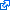# 1 paper accepted to IEEE TNSE

Our paper  on synchronization of phase-coupled oscillators was accepted to appear in IEEE Transactions on Network Science and EngineeringA. Gushchin, E. Mallada, and A. Tang, “Phase-coupled oscillators with plastic coupling: Synchronization and stability,” IEEE Transactions on Network Science and Engineering, vol. 3, iss. 4, pp. 240-256, 2016.

In this article we study synchronization of systems of homogeneous phase-coupled oscillators with plastic coupling strengths and arbitrary underlying topology. The dynamics of the coupling strength between two oscillators is governed by the phase difference between these oscillators. We show that, under mild assumptions, such systems are gradient systems, and always achieve frequency synchronization. Furthermore, we provide sufficient stability and instability conditions that are based on results from algebraic graph theory. For a special case when underlying topology is a tree, we formulate a criterion (necessary and sufficient condition) of stability of equilibria. For both, tree and arbitrary topologies, we provide sufficient conditions for phase-locking, i.e. convergence to a stable equilibrium almost surely. We additionally find conditions when the system possesses a unique stable equilibrium, and thus, almost global stability follows. Several examples are used to demonstrate variety of equilibria the system has, their dependence on system’s parameters, and to illustrate differences in behavior of systems with constant and plastic coupling strengths.

``````@article{gmt2016tnse,
abstract = {In this article we study synchronization of systems of homogeneous phase-coupled oscillators with plastic coupling strengths and arbitrary underlying topology. The dynamics of the coupling strength between two oscillators is governed by the phase difference between these oscillators. We show that, under mild assumptions, such systems are gradient systems, and always achieve frequency synchronization. Furthermore, we provide sufficient stability and instability conditions that are based on results from algebraic graph theory. For a special case when underlying topology is a tree, we formulate a criterion (necessary and sufficient condition) of stability of equilibria. For both, tree and arbitrary topologies, we provide sufficient conditions for phase-locking, i.e. convergence to a stable equilibrium almost surely. We additionally find conditions when the system possesses a unique stable equilibrium, and thus, almost global stability follows. Several examples are used to demonstrate variety of equilibria the system has, their dependence on system's parameters, and to illustrate differences in behavior of systems with constant and plastic coupling strengths.},
author = {Gushchin, Andrey and Mallada, Enrique and Tang, Ao},
doi = {10.1109/TNSE.2016.2605096},
journal = {IEEE Transactions on Network Science and Engineering},
keywords = {Synchronization},
month = {09},
number = {4},
pages = {240-256},
title = {Phase-coupled oscillators with plastic coupling: Synchronization and stability},